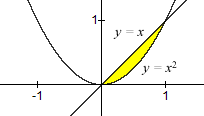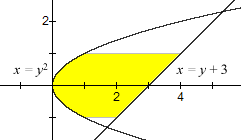index: click on a letter A B C D E F G H I J K L M N O P Q R S T U V W X Y Z A to Z index index: subject areas numbers & symbols sets, logic, proofs geometry algebra trigonometry advanced algebra & pre-calculus calculus advanced topics probability & statistics real world applications multimedia entrieswww.mathwords.com about mathwords website feedback

Area between Curves

The area between curves is given by the formulas below.

 Formula 1: Area = $$\int_a^b {\,\,\left| {f\left( x \right) - g\left( x \right)} \right|\,\,\,dx}$$ for a region bounded above by y = f(x) and below by y = g(x), and on the left and right by x = a and x = b. Formula 2: $$\int_c^d {\,\,\left| {f\left( y \right) - g\left( y \right)} \right|\,\,\,dy}$$ for a region bounded on the left by x = f(y) and on the right by x = g(y), and above and below by y = c and y = d. Example 1:1 Find the area between y = x and y = x2 from x = 0 to x = 1.\eqalign{{\rm{Area}} &= \int_0^1 {\left| {x - {x^2}} \right|dx} \\ &= \int_0^1 {\left( {x - {x^2}} \right)dx} \\ &= \left. {\left( {\frac{1}{2}{x^2} - \frac{1}{3}{x^3}} \right)} \right|_0^1\\ &= \left( {\frac{1}{2} - \frac{1}{3}} \right) - \left( {0 - 0} \right)\\ &= \frac{1}{6}} Example 2:1 Find the area between x = y + 3 and x = y2 from y = –1 to y = 1.\eqalign{{\rm{Area}} &= \int_{ - 1}^1 {\left| {y + 3 - {y^2}} \right|dy} \\ &= \int_{ - 1}^1 {\left( {y + 3 - {y^2}} \right)dy} \\ &= \left. {\left( {\frac{1}{2}{y^2} + 3y - \frac{1}{3}{x^3}} \right)} \right|_{ - 1}^1\\ &= \left( {\frac{1}{2} + 3 - \frac{1}{3}} \right) - \left( {\frac{1}{2} - 3 + \frac{1}{3}} \right)\\ &= \frac{{16}}{3}}